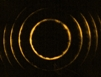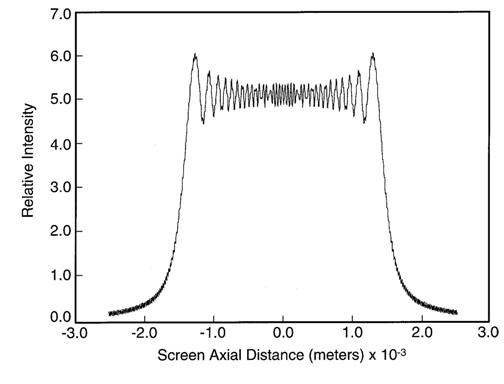Interferometric Optics

Generalized Diffractive Calculations

Numerical calculations, near or far field, for the propagation of Gaussian beams via narrow or wide apertures. Input parameters include: wavelength, aperture width, and propagation distance in metric units. Output is provided in graphical and/or numerical form.Note: the diffraction calculations mentioned here are performed using equations derived from the application of Dirac's notation to propagation in a given geometry. The principles of this approach are described in F. J. Duarte, Tunable Laser Optics, 2nd Edition (CRC, New York, 2015) (see Chapter 2). Examples of diffractive calculations induced by wide apertures, in the near field, are illustrated in Chapters 2 and 7. This particular near field diffraction distribution corresponds to Fig. 2.11 of Tunable Laser Optics.

Contact

Published on the 15th of June, 2006; updated on the 31st of January, 2020.